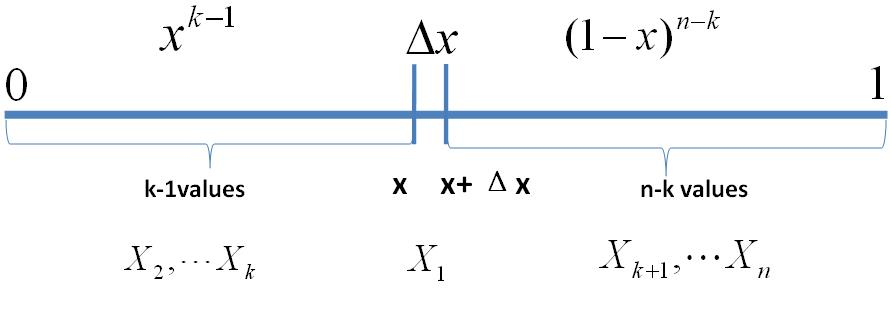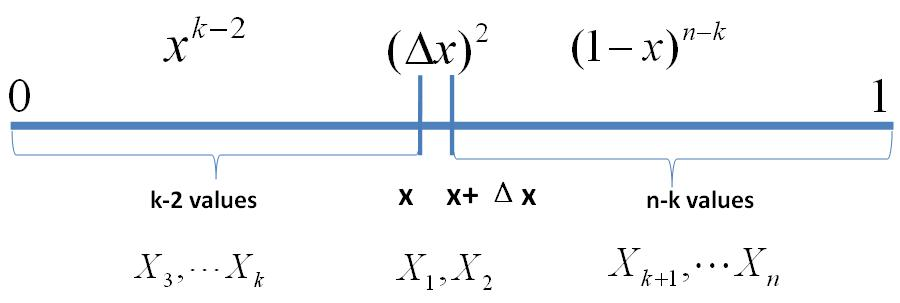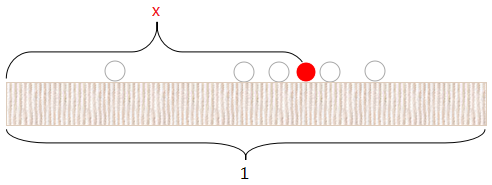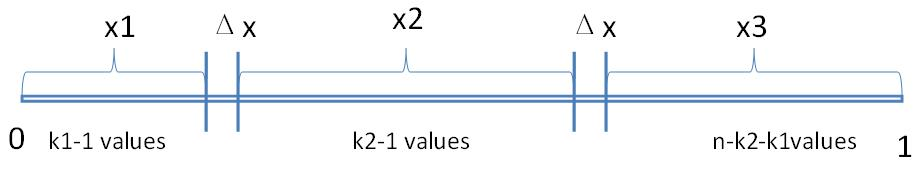# 推导

## 第一种

$\,\mathrm{Beta}\,$分布一般被用于建模伯努利试验事件成功的概率的概率分布. 什么叫做伯努利试验事件成功的概率? 我们来举个例子.

$\,x = a, n-x=b, B(a+1,b+1)=k^{-1}\,$, 也就是说

## 第二种

• 把这$\,n\,$个随机变量排序后得到顺序统计量$\,X_{(1)},X_{(2)},\cdots,X_{(n)},\,$
• $\,X_{(k)}\,$的分布是什么

$\,[0,1]\,$区间分为$\,3\,$段, 即$\,[0,x),[x,x+\Delta x],(x+\Delta x,1]\,$. 假设$\,X_{(k)}\,$在区间$\,[x,x+\Delta x]\,$中, 我们先考虑一个简单情形:

• $\,[x, x+\Delta x]\,$中只有一个点• $\,[x, x+\Delta x]\,$中有两个点$\,k=a,n-k+1=b\,$, 上式可写为

$\,\frac{1}{B(a,b)}x^{a-1}(1-x)^{b-1}\,$即为$\,Beta\,$分布的概率密度函数.

# Beta 函数与 Gamma 函数的关系$\,k=a-1,n-k=b-1\,$, 根据$\,\mathrm{Gamma}\,$函数的定义, 有

# Beta-Binomial 共轭

• $\,X_1,X_2,\cdots,X_n\mathop\sim\limits^{\mathrm{iid}}Uniform(0,1)\,$, 排序后对应的顺序统计量为$\,X_{(1)}, X_{(2)},\cdots,X_{(n)}\,$,
• $\,Y_1,Y_2,\cdots,Y_m\mathop\sim\limits^{\mathrm{iid}}Uniform(0,1)\,$, 且有$\,m_1\,$个比$\,X_{(k)}\,$大,$\,m_2\,$个比$\,X_{(k)}\,$小,
• $\,p = X_{(k)}\,$, 求$\,P(p\mid Y_1,Y_2,\cdots,Y_m)\,$的分布

# Dirichlet 分布

$\,\mathrm{Dirichlet}\,$分布其实就是多维的$\,\mathrm{Beta}\,$分布. 继续上面的游戏, 我可以说:

• 把这$\,n\,$个随机变量排序后得到顺序统计量$\,X_{(1)},X_{(2)},\cdots,X_{(n)},\,$
• $\,X_{(k_1)}\,$$\,X_{(k_1+k_2)}\,$的联合分布是什么$\,k_1=\alpha_1,k_2=\alpha_2,n-k_1-k_2+1=\alpha_3\,$